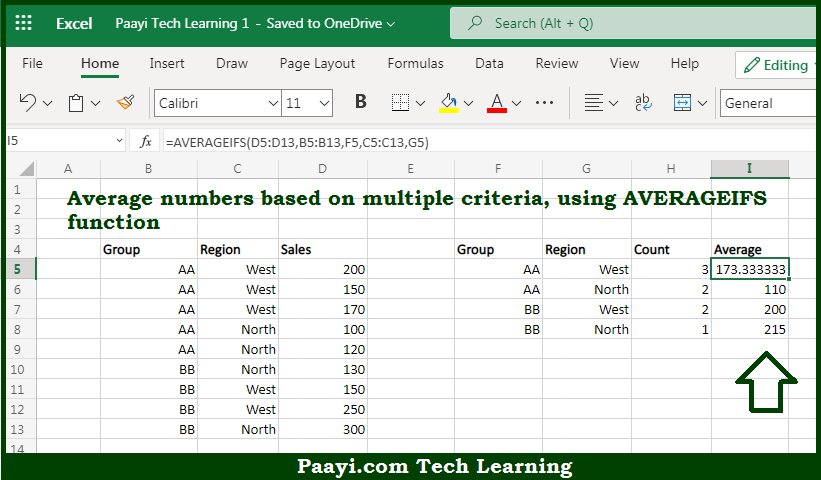# Learn How to AVERAGE With Multiple Criteria in Microsoft Excel

Written by | 0 Comments | 505 Views

In this article, you will learn how to AVERAGE With Multiple Criteria in Microsoft Excel using a single/combination(s) of functions. You will also know how to AVERAGE With Multiple Criteria and see the generic formula.

AVERAGE With Multiple Criteria in Microsoft Excel

The main purpose of this formula is to average numbers based on multiple criteria. Here we will learn how to get the average of given data range based on the multiple criteria in the workbook in Microsoft Excel. That implies, with the help of a formula based on the AVERAGEIFS function you can able to average numbers based on multiple criteria. So, with the help of this formula, you can able to get the average of a given data range based on the multiple criteria in the workbook in Microsoft Excel.

General Formula to AVERAGE With Multiple Criteria

=AVERAGEIFS(values,range1,criteria1,range2,criteria2)

The Explanation for the AVERAGE With Multiple CriteriaSo we know that with the help of the given formula above you can able to average numbers based on multiple criteria. Here we will learn how to get the average of given data range based on the multiple criteria in the workbook in Microsoft Excel. As we know that the AVERAGEIFS function is designed to handle multiple criteria which are entered in (range, criteria) pairs. If all the information is supplied correctly, the behavior of AVERAGEIFS is fully automatic. So, with the help of this formula, you can able to get the average of a given data range based on the multiple criteria in the workbook in Microsoft Excel.